# ieCivil

Engineering the Digital Civilization

## Monday, 5 October 2015

Contour project is one of the most important project in syllabus of Savitribai Phule Pune University. It requires a lot of calculation and its a very hectic work and requires hours of calculations after taking the readings. These calculations can be done within an hour using Microsoft Excel, all you need to do is make the table and then put formulas in the respective columns and then just type the values that are observed on site. Follow the steps that are mentioned below:

The first step in any survey project, while performing this step be cautious and remember to take the smallest of details possible to make the project detailed and accurate. For this Preparing a custom observation sheet is better option than carry a field book which is difficult to manage on the observation scenario. Click here to download a custom observation page which you can print and fill in the readings on site using the theodolite. Fill in the accurate and required readings and then get on the computer to calculate the values required to plot the contour layout.

2) Calculate using Microsoft Excel:

A. Start and Prepare the Environment for Calculations on Excel

Start Microsoft Excel and then prepare the table for calculations on excel. Include the columns of data that you have and columns of data that you want to calculate. After that prepare an excel sheet which is as according to the image below. You can include additional fields like the remarks, etc.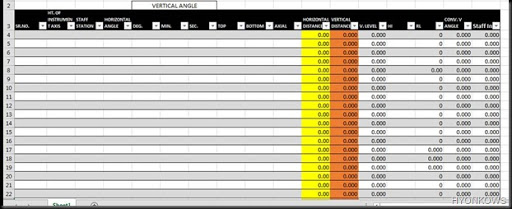Fill in the formulas under the heads of columns as required: After preparing the table it is required to fill the formulas and then start feeding the values and calculating the required values. As the survey was carried out using 20” theodolite we get the angles with the accuracy up to 20” (seconds). So we need to calculate the values up to that precision. To calculate the values follow the steps:
i) Convert the Angle: The angle we get by observation on field is in Degree, Minute and Seconds like for example in the following format 10°28’40” but inserting these values in excel is a task which is not possible so it is better that we convert that angle in to value which can be utilised for further calculations like 10°28’40” can be converted to 10.478 using excel. For this I prefer creating a column which has the formula for converting minutes and seconds value to it corresponding degree value and then adding all the three for getting the converted vertical angle. Refer the image below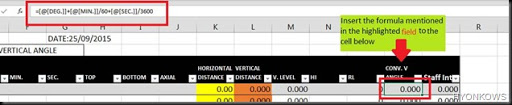Now for excel to convert the minutes and seconds into its degree equivalent it is essential that we feed the degree minutes and seconds in separate cells as visible in the image above. You don’t need to pule the formula in every cell, instead once you feed the formula in one cell of a table it its taken as common formula for all the rows of the table if its not happening then you can use the over-write option from the drop down list. Once you’re done with this step you can proceed with the next step that is calculation of horizontal distance.

ii) Horizontal Distance/Component: in tachometric contour survey you don’t need to measure the distance using the tape or all you need is the value of top, bottom, axial and the horizontal angle of inclination. Using the formula you can easily calculate the horizontal distance between the staff station and the instrument. Use the formula mentioned the formula field of the image below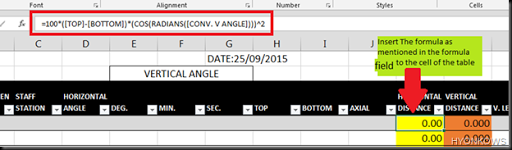Use the formula as it is in the field a minor change can cause a great variation in your value.

iii) Vertical Component: This is the value that portrays the vertical displacement of the staff that is in up or down direction like for a place you’ll get the value as 4.655 and at a place you’ll get the value as 1.240 also note that when the vertical angle is changing the value of vertical component also changes the magnitude of vertical component depends upon the values of Top, Bottom, Axial and Vertical angle.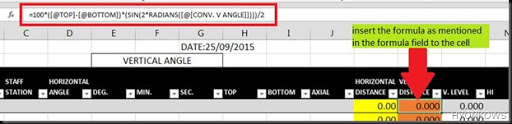As visible in the formula the vertical component Is a sine function of the vertical angle and so if the angle Is 0° then you’ll get the value as 0 but this not possible. What I’ve observed is that when the vertical angle is zero the theodolite acts as an Autolevel and its axial reading should be the staff reading so for that purpose I’ve added another field as V.LEVEL in which I’ve used the IF function of excel which takes the value of axial reading as the vertical component when the angle is zero else it gives the same value as of its preceding cell.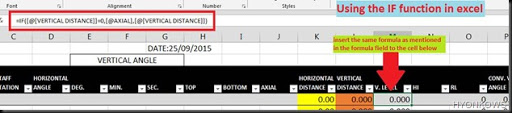Use the IF function of excel for calculating the correct level of the station.
iv) Reduced Levels: The main objective of almost all the survey projects is to calculate the RL of stations with respect to some benchmark. This can even done on excel. While calculating the RL on excel I personally prefer the HI (Height of Instrument) Method as it reduces the effort of typing formulas and you can just drag the cell down to copy the values making it an easy task.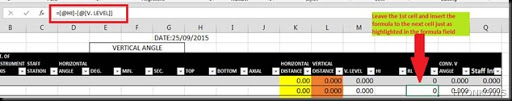Now leaving the 1st cell and inserting the formula to the next cell makes it complete. All you need to do is type the RL of benchmark in the 1st cell and type the HI by adding the RL and V.Level Value in the HI Column, drag the HI cell to below so that you can copy the value of the HI at benchmark for calculation of HI at staff stations.
You can use the conditional formatting to compare values and find out highest and lowest RL and also use formatting for identification of cells to avoid confusion.
Finally you have all the values required to plot the contour layout. Saving a lot of time and efforts and over-all is the accuracy of results as manual error is negligible in this procedure as compared to the manual calculations.